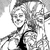## FANDOM

25,366 Pages# Alakabamm

0 Discussion posts
•## Durability Required to be Completely Bulletproof

August 13, 2016 by Alakabamm

So this blog attempts to answer an interesting question: at what level do characters become completely immune to conventional bullets? Insofar as VSBW goes, this is not very useful because most weapons act far more powerful than they really should be and/or are magical, but it could be useful if comparing certain characters to real life statements.

Note that because this wiki considers durability to apply equally over the body, I will simply be using the surface area of a human body and comparing it to that of a bullet tip. However, it is entirely possible for certain points of the body to have lesser durability, such as the eyes, so keep that in mind as well.

The largest caliber round in the world is the 0.950 JDJ. This gives us a good high…

•## Vaporization of Titanium Value

August 11, 2016 by Alakabamm

Since we don't have a source for this, I will just do it quickly myself.

One cubic centimeter of titanium would have a mass of 4.506 grams.

From that same link, it has a boiling point of 3287 Celsius, a heat of fusion of 14,500 Joules/mol, a heat of vaporization of 425,000 Joules/mol and a molar heat capacity of 25.060 J/(mol*C)

Using the molar mass of titanium (47.867 g/mol), we convert the heat of fusion, heat of vaporization and molar heat capacity to be 302.922 Joules/gram, 8878.768 Joules/gram and 0.5235 J/gram*C.

Using the specific heat equation, we find the energy change to be (assuming room temperature) = (4.506)(0.5235 J/gram*C)(3287-20) = 7706.997 Joules.

Using the latent heat equation (as linked above as well), we find the other ener…

•## The Invincible Superman Tanks a Missile (Kenichi)

July 30, 2016 by Alakabamm

So, at the end of the Kenichi manga, the elder "sacrifices" his life to take on a missile...and comes back without even a scratch.

So let's estimate how powerful this thing was.

Scaling 1:

Hayato = 2m (at least) = 81 pixels

Rocket width = 288 pixels = 7.11 m

Scaling 2:

Rocket trail (should be approximately equal to rocket width) = 7.11 m = 11 pixels

Explosion width = 601 pixels = 388.5 m

Plugging into the nuke calculator, we get the fireball at the correct range with a yield of 0.082 megatons of TNT or 82 kilotons of TNT

Halving because we are using the nuclear explosion calc, the true value should be: 42 kilotons of TNT or Town level.

Edit:

If near-total fatalities is preferred instead: Air blast radius (near-total fatalities) Yield: Y=((x/0.28)^3)/1…

•## Energy Required to Atomize New York State (Rough)

July 30, 2016 by Alakabamm

In this blog post, I will take a look at the energy needed to atomize the entire state of new york, not just the city.

To start with, the area of new york as a state is 141,300 km^2. So this will adequately fulfill two of the three dimensions required for the energy calc.

As for what substance to use, it is dependent on the method; in this case, I will be using difference in elevation as the third dimension (across the state) so rock should suffice. Hence, this calc will not be looking at the (possibly) more minor effects of atomizing New York State (i.e. everything on the surface), but rather the entirety of the rock itself (to a degree).

According to this site, New York average elevation varies from 1000 feet to sea level (0 feet). So our t…

•## Call of Duty Calc

July 30, 2016 by Alakabamm

Originally calculated here

Feat

Scaling:

Crate stack height = 243 pixels = 1.22m

Screen height = 1080 pixels

Angsizing for degrees, degrees = 2atan(tan(70/2)*(243/1080)) = 8.95 degrees

Plugging into angsize calculator gives us a distance of 7.7943 meters. Timeframe of travel is 1 second, so speed = 7.7943m/s.

Plugging into KE (1/2mv^2) and assuming american tons, we get a total energy of 5511.2 joules.